05/30 11:37

## 写在前面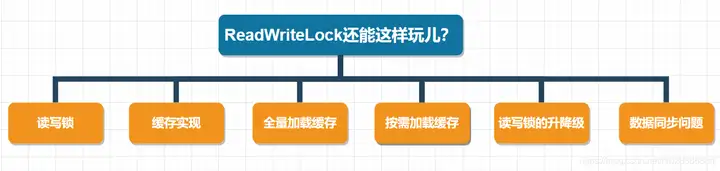## 读写锁

• 一个共享变量允许同时被多个读线程读取到。
• 一个共享变量在同一时刻只能被一个写线程进行写操作。
• 一个共享变量在被写线程执行写操作时，此时这个共享变量不能被读线程执行读操作。

public ReentrantReadWriteLock(boolean fair) {
sync = fair ? new FairSync() : new NonfairSync();
writerLock = new WriteLock(this);
}

## 缓存实现

public class ReadWriteLockCache<K,V> {
private final Map<K, V> m = new HashMap<>();
// 读锁
private final Lock r = rwl.readLock();
// 写锁
private final Lock w = rwl.writeLock();
// 读缓存
public V get(K key) {
r.lock();
try { return m.get(key); }
finally { r.unlock(); }
}
// 写缓存
public V put(K key, V value) {
w.lock();
try { return m.put(key, value); }
finally { w.unlock(); }
}
}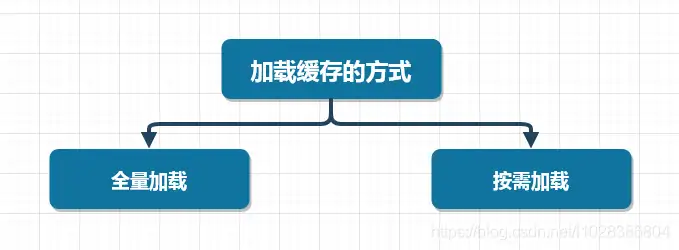## 全量加载缓存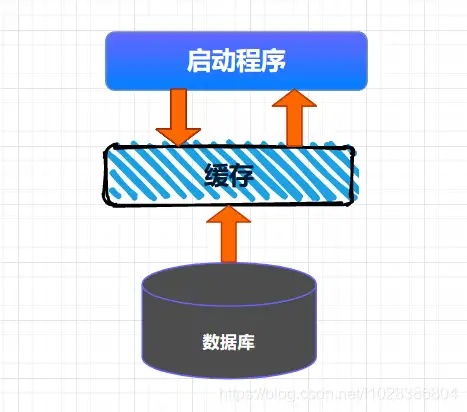public class ReadWriteLockCache<K,V> {
private final Map<K, V> m = new HashMap<>();
// 读锁
private final Lock r = rwl.readLock();
// 写锁
private final Lock w = rwl.writeLock();
//查询数据库
List<Field<K, V>> list = .....;
if(!CollectionUtils.isEmpty(list)){
list.parallelStream().forEach((f) ->{
m.put(f.getK(), f.getV);
});
}
}
// 读缓存
public V get(K key) {
r.lock();
try { return m.get(key); }
finally { r.unlock(); }
}
// 写缓存
public V put(K key, V value) {
w.lock();
try { return m.put(key, value); }
finally { w.unlock(); }
}
}

## 按需加载缓存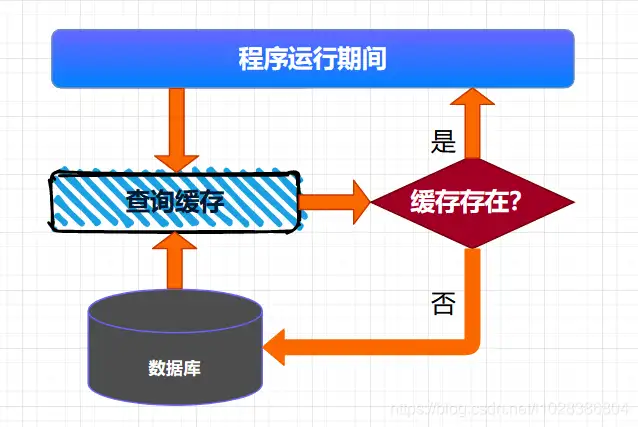class ReadWriteLockCache<K,V> {
private final Map<K, V> m = new HashMap<>();
private final Lock r = rwl.readLock();
private final Lock w = rwl.writeLock();
V get(K key) {
V v = null;
//读缓存
r.lock();
try {
v = m.get(key);
} finally{
r.unlock();
}
//缓存中存在，返回
if(v != null) {
return v;
}
//缓存中不存在，查询数据库
w.lock();
try {
//再次验证缓存中是否存在数据
v = m.get(key);
if(v == null){
//查询数据库
v=从数据库中查询出来的数据
m.put(key, v);
}
} finally{
w.unlock();
}
return v;
}
}

## 读写锁的升降级

class CachedData {
Object data;
volatile boolean cacheValid;
void processCachedData() {
if (!cacheValid) {
// Must release read lock before acquiring write lock
rwl.writeLock().lock();
try {
// Recheck state because another thread might have
// acquired write lock and changed state before we did.
if (!cacheValid) {
data = ...
cacheValid = true;
}
} finally {
rwl.writeLock().unlock(); // Unlock write, still hold read
}
}
try {
use(data);
} finally {
}
}
}}

## 数据同步问题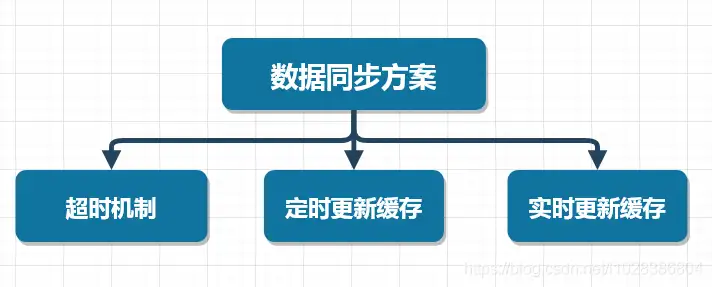## 定时更新缓存0 评论
1 收藏
0